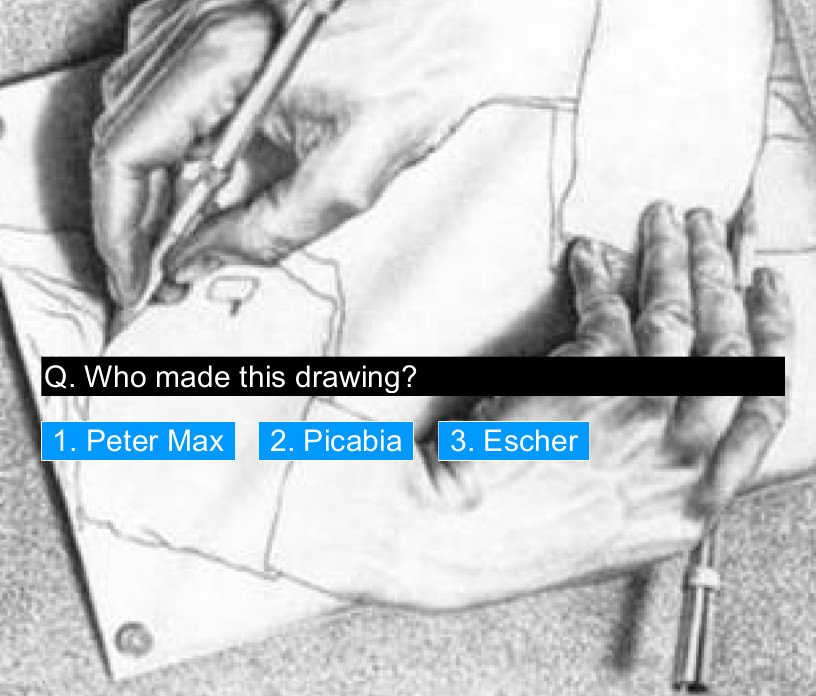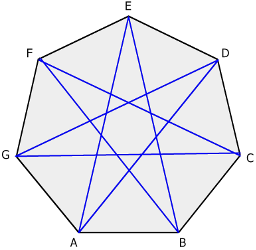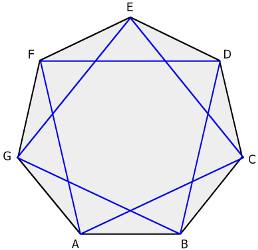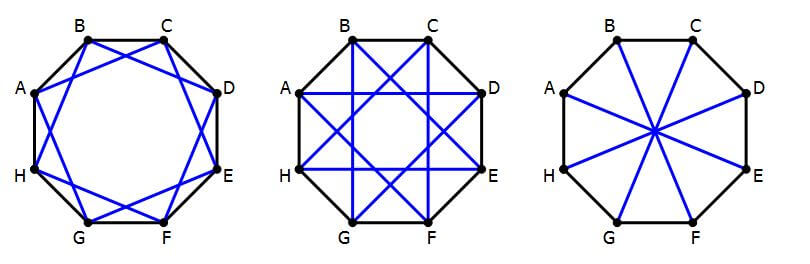# 1r ESO CLIL

UNIT1. POINT

1.1 WORKSHEET: “Dot to dot”

1.2. WORKSHEET: “Collage”

http://www.ehow.com/how_2290221_make-collage-using-newspaper.html

UNIT 2. LINES

2.1. Activity 1 ESO

At first, we give each student a blank, for 2 minutes with any tool, they must draw different types of lines on paper.

Finalized this time, students should try to name and classify the various types of lines, which will last one minute.

From this issue, not really knowing the name you have to put your lines drawn on paper.

The teacher put a video in English, so that students try to identify their lines, listening and watching the video, to put the right name to the lines and classify them.

Then, once everyone has tried to solve the problem is put together. Each student teaches his role and says the name of the lines and the rest of the class say whether it is right or not. If you have any questions, the teacher makes relevant explanations, all in English.

Finally, the teacher and students have the scorecard on the board and when everyone agrees and understands it. Students copy the summary of the board at the back of the same paper.

RECOGNIZING ARThttps://photopeach.com/album/754qii?ref=est

Recognizing Art on PhotoPeach includes a quizz

STORYBOARD

http://english.thestoryboardmethod.com/

SHAPE

TEXTURE

GEOMETRYwhorksheet 1. BASIC GEOMETRY

• Perpendicular segment line bisector

• Perpendicular line to another though an outer point of it

• Perpendicular line of a segment through an endpoint.

• Parallel line to another one through an outer point of the given one.

WORKSHEET 2. BASIC OPARETIONS WITH ANGLES (FOUR PARTS). First we will see a video about the theory of angles. Then, you will be divided the worksheet into four parts. Each part has the title and video support to do the exercise.

1. THE ANGLE’S BISECTOR

2. ANGLE TRISECTOR (Divide a straight angle into three equal parts).

3. ANGLES ADITION RULER AND COMPASS

Firts you see these video about how to copy angles geometry. then, you see the other for to resolt the exercice.

4. ANGLES SUBTRACTION WITH RULER AND COMPASS

WORKSHEET 3. DEFINITIONSS AND CLASSIFICATIONS ABOUT TRIANGLES (FOUR PARTS).

Triangle: It is a flat figure with three sides and three angles.

3.1 EQUILATERAL TRIANGLE CONSTRUCTION KNOWN ITS SIDE (5 cm)

3.2 ISOSCELES TRIANGLE CONSTRUCTION KNOWN ITS TWO SIDES: a=3cm I b=6cm.

3.3 SCALENE TRIANGLE CONSTRUCTION KNOWN ITS THREE SIDES: a=6cm, b=5cm i C=3cm.

3.4 RIGHT TRIANGLE CONSTRUCTION KNOWN ITS TWO SIDES: a=6cm I b=4cm.3.1 SQUARE CONSTRUCTION KNOWN ITS SIDE DIMENSION. l=5cm.

3.2 RECTANGLE CONSTRUCTION KNOWN THE DIAGONAL AND ONE OF ITS SIDE. d=6cm, a=3cm.

3.3 RHOMBUS CONSTRUCTION KNOWN ONE OF THE ANGLE AND THE SIDES. <60º, AB=5cm.

3.4 RIGHT TRAPEZIUM CONSTRUCTION KNOWN TWO SIDES AND THE HIGHT. a=7cm, b=5cm, h=4cm.

WORKSHEET 4. DEFINITIONSS AND CLASSIFICATIONS ABOUT POLYGONS (FOUR PARTS).

4.1 DRAW A SQUARE INSCRIBED IN A CIRCLE. r=3cm.

4.2 DRAW A REGULAR PENTAGON INSCRIBED IN A CIRCLE. r=3cm.

4.3 DRAW A REGULAR HEXAGON INSCRIBED IN A CIRCLE. r=3cm.

4.4 DRAW A REGULAR HEPTAGON INSCRIBED IN A CIRCLE. r=3cm.

Star polygons

5. WORKSHEET STAR POLYGON5.1. HOW TO MAKE A STAR CIRCUMSCRIBED IN A PENTAGON

5.2. HOW TO MAKE A STAR CICUMSCRIBED IN A HEXGON5.3. HOW TO MAKE A STAR CICUMSCRIBED IN A HEPTAGON5.4. HOW TO MAKE A STAR CICUMSCRIBED IN A OCTAGON6. WORKSHEET 12 POINTED STAR

COLOUR

Go to Top